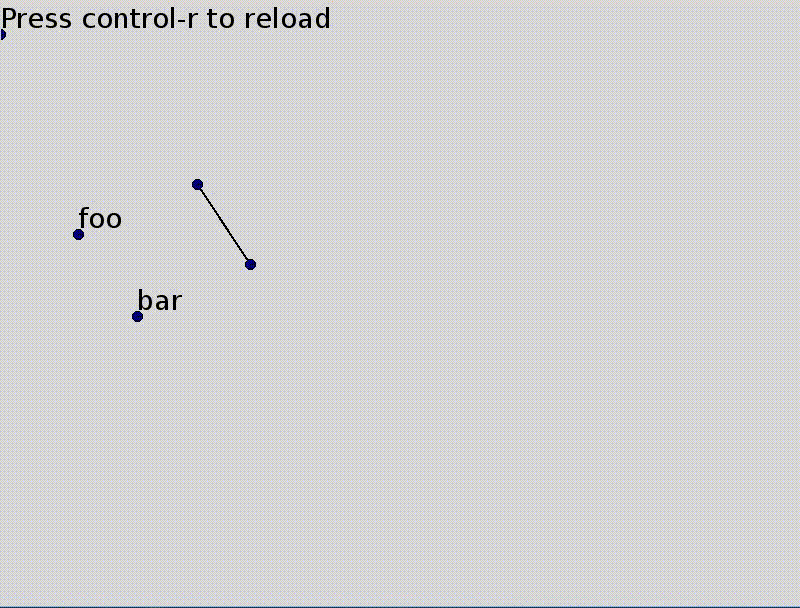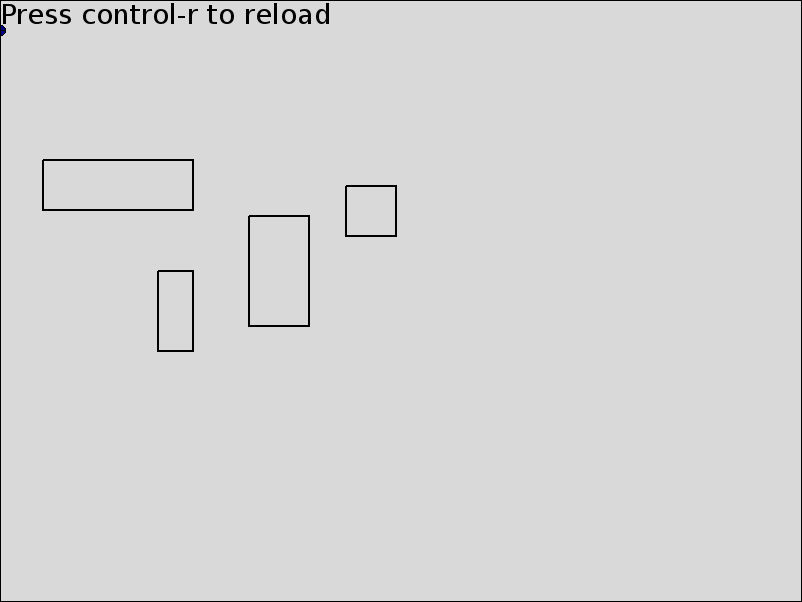# Removing polling checks in guitktk

Updating a rectangular bounding box of a selection was a bit of a hack in guitktk: every frame the selection was checked for changes and the rectangle recreated if needed. In this post, I'll show how to remove that check using "formulas" for parameter values.

In guitktk, when a selected item changed, the selection rectangle is updated.Previously, a new rectangle was created and replaced the old rectangle like so.

``````children = [
Node("line", p_start=upper_left, p_end=(lower_right, upper_left)),
Node("line", p_start=(lower_right, upper_left), p_end=lower_right),
Node("line", p_start=lower_right, p_end=(upper_left, lower_right)),
Node("line", p_start=(upper_left, lower_right), p_end=upper_left)]
old_rectangle.replace(children)
``````

The same happened for the large rectangle containing all selections.

``````if changed:
if len(self["selection"]) <= 1:
self["selection_bbox"].replace([])
else:
upper_left, lower_right = self["selection"].bbox(self["selection"].combined_transform())
children = [
Node("line", p_start=upper_left, p_end=(lower_right, upper_left)),
Node("line", p_start=(lower_right, upper_left), p_end=lower_right),
Node("line", p_start=lower_right, p_end=(upper_left, lower_right)),
Node("line", p_start=(upper_left, lower_right), p_end=upper_left)]
self["selection_bbox"].replace(children)
self["selection"].updated = time.time()
``````

While this worked well, this code polls the section every frame and feels out of place. One way around this would be to add callbacks but we'll do a bit better than that.

To avoid this, we'll make use of formulas

``````def rectangle(**params):
newnode = Node("path", skip_points=True,
p_topleft=exr("`self.parent.corners"),
p_botright=exr("`self.parent.corners"),
topright=exr("topright(`self.corners, (`self.parent).transform)"),
botleft=exr("botleft(`self.corners, (`self.parent).transform)"),
children=[
Node("line", start=exr("`self.parent.topleft"),
end=exr("`self.parent.topright")),
Node("line", start=exr("`self.parent.topright"),
end=exr("`self.parent.botright")),
Node("line", start=exr("`self.parent.botright"),
end=exr("`self.parent.botleft")),
Node("line", start=exr("`self.parent.botleft"),
end=exr("`self.parent.topleft")),],
**params)
return newnode
``````

This `rectangle` function is only called once during initialization and is the only child of this `selection_bbox` group

``````Node("group", id="selection_bbox",
stroke_color=(0.5, 0, 0), dash=([5,5],0), skip_points=True,
children=[rectangle(corners=exc("(`selection).bbox()"))])
``````

`exc` and `exr` create formulas/expressions with different reevaluation strategies [recalc]. When a selected object is altered, the node with id `selection` changes values causing the `corners` parameter of the `selection_bbox`'s child. `corners` is set to the selection bounding box's corners as a tuple of arrays (something like, `(array([0, 0]), array([100, 200])`).

Then parameters of the child that depend on `corners` are recalculated [recalc]. They are `p_topleft`, `topright`, `p_botright` and `botleft`. Then, since these values have change, the start and end points of the four `line`s in this `path` are updated.

But what happens if no element is selected. Then `(`selection).bbox()` evaluates to `(None, None)` and a lot of other values would evalute to `None` giving many errors when drawn.

Instead, we'll make the `selection_bbox` group invisible when that happens. In fact, we'll also make it invisible when only one element is selected. This is done by setting is `visible` parameter to the formula `exc("len(`selection) > 1")`.

``````Node("group", id="selection_bbox",
stroke_color=(0.5, 0, 0), dash=([5,5],0), skip_points=True,
children=[rectangle(corners=exc("(`selection).bbox()"),,
visible=exc("len(`selection) > 1"))]),
``````

Now we have the same outcome as before without polling.

We can then use minor variations of the `rectangle` function to draw rectangles.

``````def rectangle2(**params):
return Node("path",
corners=exr("(`self.topleft.value, `self.botright.value)"),
topright=exr("topright(`self.corners, (`self.parent).transform)"),
botleft=exr("botleft(`self.corners, (`self.parent).transform)"),
children = [
Node("line", start=exr("`self.parent.topleft"),
end=exr("`self.parent.topright")),
Node("line", start=exr("`self.parent.topright"),
end=exr("`self.parent.botright")),
Node("line", start=exr("`self.parent.botright"),
end=exr("`self.parent.botleft")),
Node("line", start=exr("`self.parent.botleft"),
end=exr("`self.parent.topleft")),
], **params)

doc["drawing"].append(rectangle2(p_topleft=doc["editor.mouse_xy"],
p_botright=doc["editor.mouse_xy"] + P(50, 50)))
``````## Footnotes

[recalc] The time at which recalculation actually occurs varies. `exr` formulas are (always) reevaluated when the parameter is read. `Ex` formulas are recalculated when the terms they depend on change value and that value is cached when reading. `exc` formulas are like `Ex` but their cache is initialized at the first read (after a value changes).